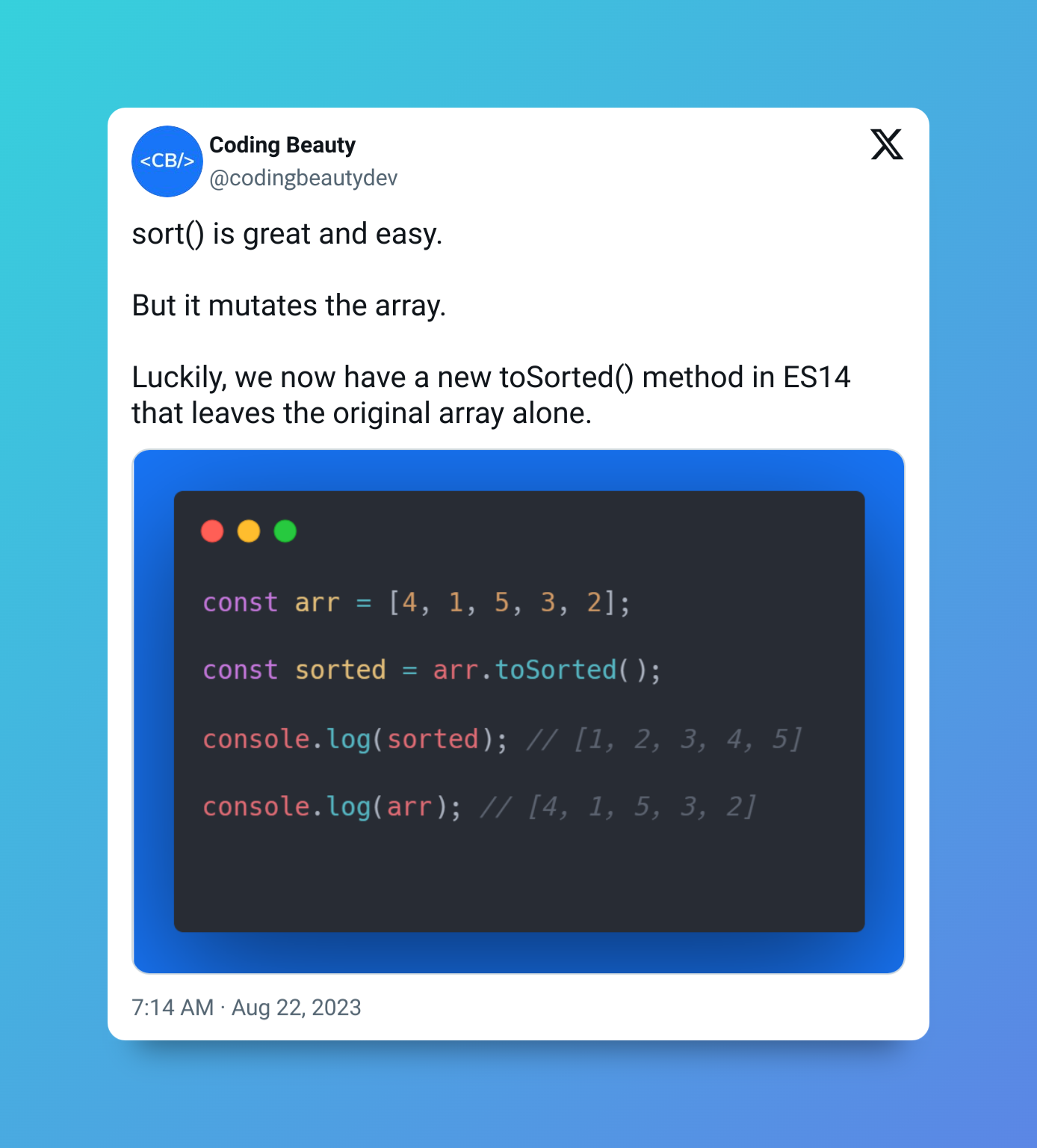# 3 Easy Ways to Swap 2 Array Elements in JavaScript

In this article, we’ll learn multiple ways to easily swap the values of two array elements in JavaScript.### 1. Temporary Variable

To swap two array elements in JavaScript:

1. Create a temporary variable to store the value of the first element.
2. Set the first element to the value of the second element.
3. Set the second element to value in the temporary variable.

For example:

``````function swapElements(arr, i1, i2) {
// Step 1
let temp = arr[i1];

// Step 2
arr[i1] = arr[i2];

// Step 3
arr[i2] = temp;
}

const arr = [1, 2, 3, 4];

swapElements(arr, 0, 3);

console.log(arr); // [ 4, 2, 3, 1 ]
``````

### 2. Array Destructuring Assignment

To swap two array elements with this method:

1. Create a new array, containing both elements in a particular order.
2. Use the JavaScript array destructing syntax to unpack the values from the array into a new array that contains both elements in a reversed order.

With this method, we can create a concise one-liner to do the job.

``````function swapElements(arr, i1, i2) {
[arr[i1], arr[i2]] = [arr[i2], arr[i1]];
}

const arr = [1, 2, 3, 4];

swapElements(arr, 0, 3);

console.log(arr); // [ 4, 2, 3, 1 ]
``````

### 3. Array splice() Method

An alternative approach to swapping two array elements is to use the `splice()` method, like this:

``````function swapElements(arr, i1, i2) {
arr[i1] = arr.splice(i2, 1, arr[i1]);
}

const arr = [1, 2, 3, 4];

swapElements(arr, 0, 3);

console.log(arr); // [ 4, 2, 3, 1 ]
``````

The `Array` `splice()` method changes the contents of an array by removing existing elements, while possibly adding new elements in their place.

``````const arr1 = ['a', 'b', 'c'];

// Removing elements
arr1.splice(1, 2);
console.log(arr1); // [ 'a' ]

// Removing and adding new elements
const arr2 = ['a', 'b', 'c'];
arr2.splice(1, 2, 'd', 'e');
console.log(arr2); // [ 'a', 'd', 'e' ]``````

In order to swap the array elements, we specify certain arguments for three of the parameters that `splice()` has:

1. `start`: This specifies the index from which to start changing the array from. We use the index of the second element for this as the argument for the parameter.
2. `deleteCount`: This is a number that indicates the number of elements to remove from `start`. Our passing `1` means that only the element at the `start` index (the second element to be swapped) will be removed.
3. `item1`, …, `itemN`: These are a variable number of arguments to add to the array. We only pass one value for this – the first element to be swapped.

So the three arguments we pass to `splice()` replace the first element with the second.

To complete the swap, we take advantage of the fact that `splice()` returns an array containing removed elements.

``````const arr2 = ['a', 'b', 'c'];

const removed = arr2.splice(1, 2, 'd', 'e');

console.log(removed); // [ 'b', 'c' ]
console.log(arr2); // [ 'a', 'd', 'e' ]
``````

So for our scenario, the second element to be swapped will be in this array, hence we access it from the array and assign its value to the first element.

## Every Crazy Thing JavaScript Does

A captivating guide to the subtle caveats and lesser-known parts of JavaScript.